• 基于spss的一元线性回归与多元线性回归案例，个人整理出的，包含了部分案例、实验报告、题目，及部分题目答案，适合作为spss、MATLAB等软件数据分析题目联系
• 今天小编就为大家分享一篇sklearn+python:线性回归案例，具有很好的参考价值，希望对大家有所帮助。一起跟随小编过来看看吧
• 案例是以一个房价面积预测房屋价格的数据为例，使用Python进行的线性模型的创建。适合新手练手。
• ML之LiR：基于编程实现简单线性回归案例 目录 LiR算法思路配图 编程实现简单线性回归案例 LiR算法思路配图 1、LiR输出结果 编程实现简单线性回归案例 结果显示 ...
ML之LiR：基于编程实现简单线性回归案例

目录
LiR算法思路配图
编程实现简单线性回归案例

LiR算法思路配图
1、LiR输出结果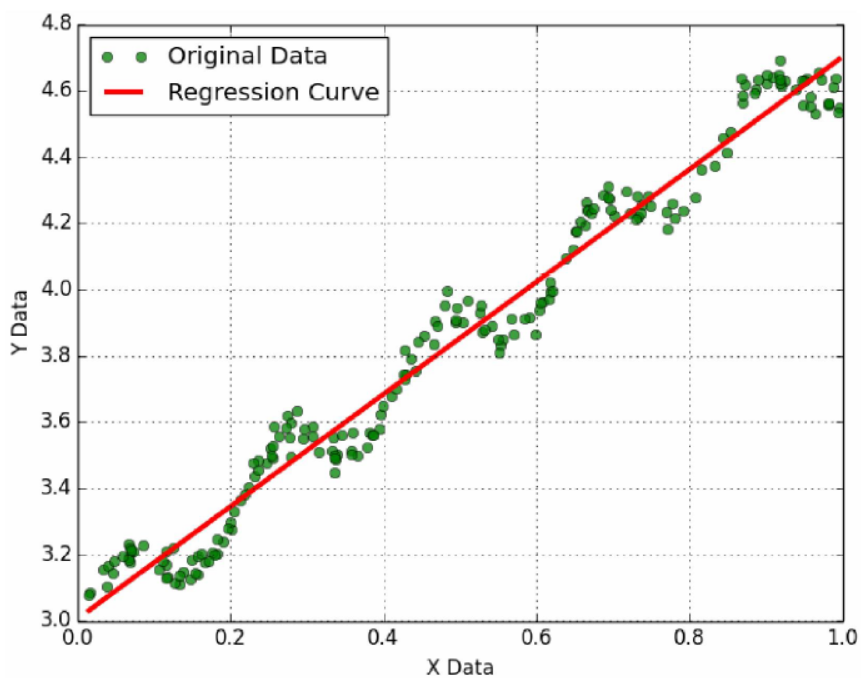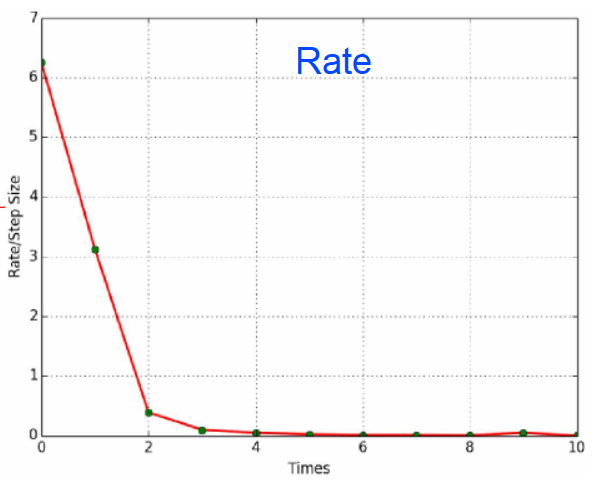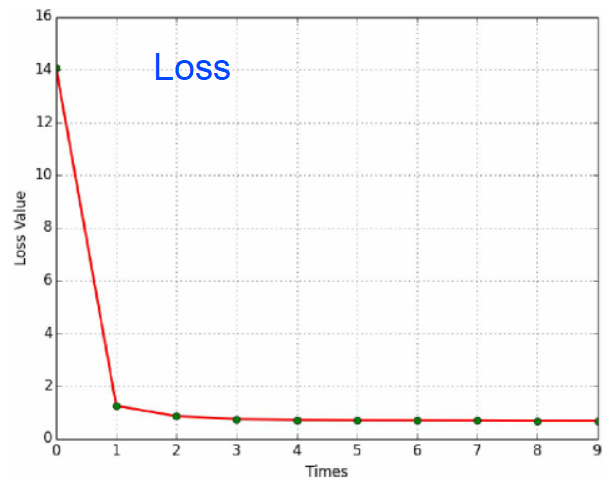编程实现简单线性回归案例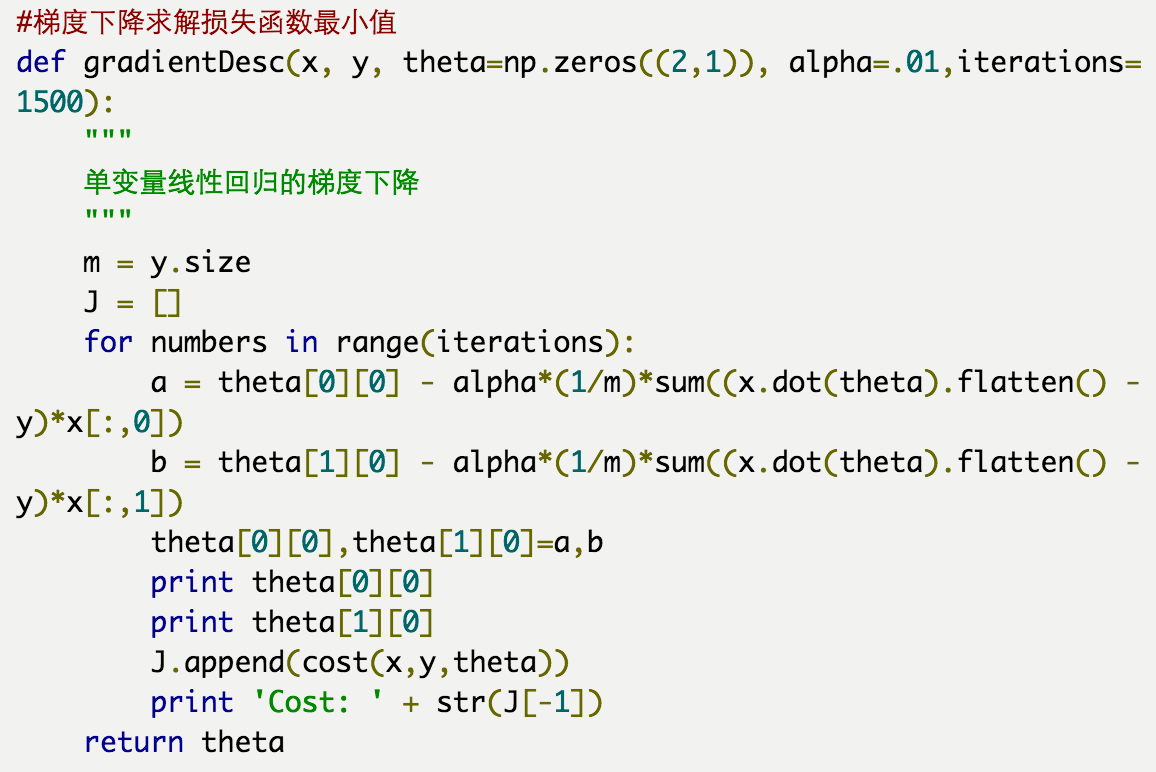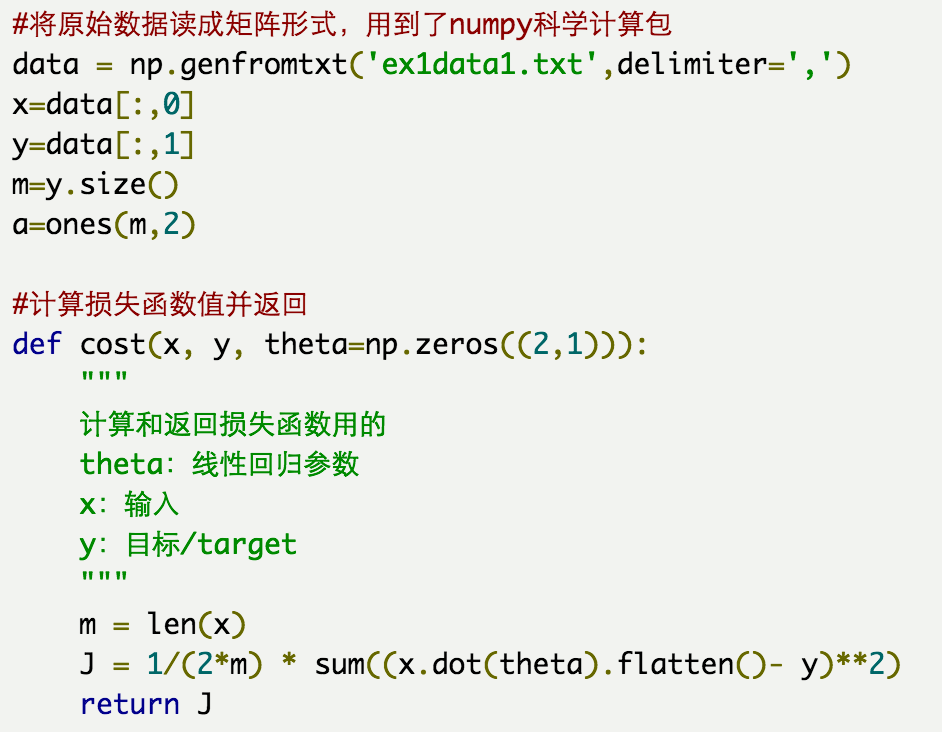结果显示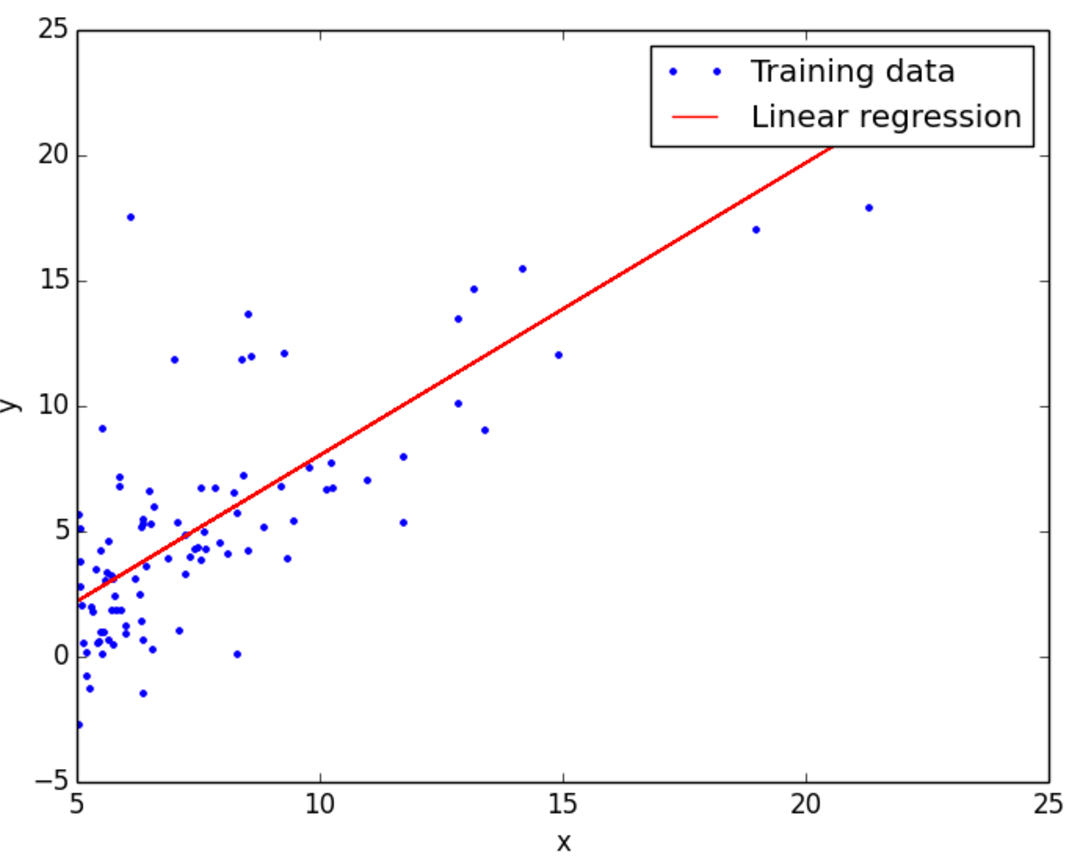展开全文• 通过具体的案例讲解时间序列下多元线性回归在eviews里的操作
• 基于EXCEL的一元线性回归案例：广告与销量基于EXCEL的一元线性回归案例：广告与销量业务分析一元线性回归步骤业务解读 基于EXCEL的一元线性回归案例：广告与销量 一元线性回归是分析只有一个自变量（自变量x和因变量...


基于EXCEL的一元线性回归案例：广告与销量
CPDA案例：基于EXCEL的一元线性回归案例《广告与销量》业务分析一元线性回归步骤业务解读

CPDA案例：基于EXCEL的一元线性回归案例《广告与销量》
一元线性回归是分析只有一个自变量（自变量x和因变量y）线性相关关系的方法。一个经济指标的数值往往受许多因素影响，若其中只有一个因素是主要的，起决定性作用，则可用一元线性回归进行预测分析。
业务分析
数据如下图所示，需要分析广告费用对销售收入的影响。一元线性回归步骤
1、选择模型： 在EXCEL的数据分析中选择回归。2、确定自变量和因变量 本案例是分析广告费用对销售收入的影响，因此广告费用为自变量Y，销售收入为因变量X。3、输出结果，参数检验 结果如下图所示。相关系数MultipleR=0.93,强相关。拟合优度R^2>0.8。 F检验：Significance F=P-value<0.05，模型成立。 该案例的一元线性回归方程为： Y=274.55+5.13X ,正相关。业务解读
广告投入和销售的收入正相关，不投入广告，基础的销售收入为274.55万元。每投入1万元的广告，收入增加5.13万元。
展开全文机器学习 大数据 数据分析 算法
• 1. tensorflow实现简单的线性回归案例 1.1 线性回归知识复习 1.2 相关API import tensorflow as tf import os os.environ['TF_CPP_MIN_LOG_LEVEL']='2' def myregression(): """ 自实现一个线性回归预测 :...
1. tensorflow实现简单的线性回归案例
1.1 线性回归知识复习1.2 相关APIimport tensorflow as tf
import os
os.environ['TF_CPP_MIN_LOG_LEVEL']='2'

def myregression():
"""
自实现一个线性回归预测
:return: None
"""
# 1、准备数据，x 特征值 [100, 1]   y 目标值
x = tf.random_normal([100, 1], mean=1.75, stddev=0.5, name="x_data")

# 矩阵相乘必须是二维的
y_true = tf.matmul(x, [[0.7]]) + 0.8

# 2、建立线性回归模型 1个特征，1个权重， 一个偏置 y = x w + b
# 随机给一个权重和偏置的值，让他去计算损失，然后再当前状态下优化
# 用变量定义才能优化
weight = tf.Variable(tf.random_normal([1, 1], mean=0.0, stddev=1.0), name="w")
bias = tf.Variable(0.0, name="b")

y_predict = tf.matmul(x, weight) + bias

# 3、建立损失函数，均方误差
loss = tf.reduce_mean(tf.square(y_true - y_predict))

# 4、梯度下降优化损失 leaning_rate: 0 ~ 1, 2, 3,5, 7, 10

# 定义一个初始化变量的op
init_op = tf.global_variables_initializer()

# 通过会话运行程序
with tf.Session() as sess:
# 初始化变量
sess.run(init_op)

# 打印随机最先初始化的权重和偏置
print("随机初始化的参数权重为：%f, 偏置为：%f" % (weight.eval(), bias.eval()))

# 循环训练 运行优化
for i in range(100):

sess.run(train_op)

print("第%d次优化的参数权重为：%f, 偏置为：%f" % (i, weight.eval(), bias.eval()))

return None

if __name__ == "__main__":
myregression()


输出的结果为：展开全文tensorflow 深度学习
• 线性回归案例分析线性回归案例分析波士顿房价预测 4.2. 线性回归案例分析 线性回归案例分析 波士顿房价预测 使用scikit-learn中内置的回归模型对“美国波士顿房价”数据进行预测。对于一些比赛数据，可以从kaggle...


文章目录
4.2. 线性回归案例分析线性回归案例分析波士顿房价预测

4.2. 线性回归案例分析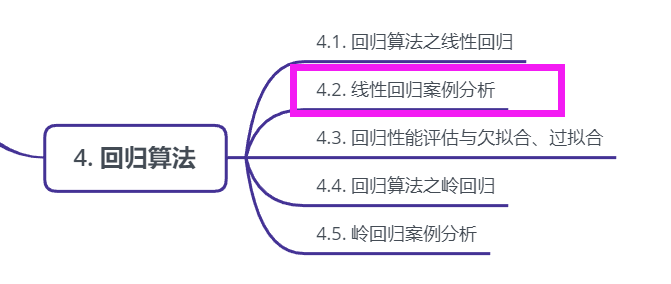线性回归案例分析
波士顿房价预测
使用scikit-learn中内置的回归模型对“美国波士顿房价”数据进行预测。对于一些比赛数据，可以从kaggle官网上获取，网址：https://www.kaggle.com/datasets
1.美国波士顿地区房价数据描述
from sklearn.datasets import load_boston

print boston.DESCR

2.波士顿地区房价数据分割
from sklearn.cross_validation import train_test_split
import numpy as np
X = boston.data
y = boston.target

X_train,X_test,y_train,y_test = train_test_split(X,y,random_state=33,test_size = 0.25)

3.训练与测试数据标准化处理
from sklearn.preprocessing import StandardScaler
ss_X = StandardScaler()
ss_y = StandardScaler()

X_train = ss_X.fit_transform(X_train)
X_test = ss_X.transform(X_test)
y_train = ss_X.fit_transform(y_train)
X_train = ss_X.transform(y_test)

4.使用最简单的线性回归模型LinearRegression和梯度下降估计SGDRegressor对房价进行预测
from sklearn.linear_model import LinearRegression
lr = LinearRegression()
lr.fit(X_train,y_train)
lr_y_predict = lr.predict(X_test)

from sklearn.linear_model import SGDRegressor
sgdr = SGDRegressor()
sgdr.fit(X_train,y_train)
sgdr_y_predict = sgdr.predict(X_test)

5.性能评测
对于不同的类别预测，我们不能苛刻的要求回归预测的数值结果要严格的与真实值相同。一般情况下，我们希望衡量预测值与真实值之间的差距。因此，可以测评函数进行评价。其中最为直观的评价指标均方误差(Mean Squared Error)MSE，因为这也是线性回归模型所要优化的目标。
MSE的计算方法如式：
{MSE=}\frac{1}{m}\sum_{i=1}{m}\left({y{i}-\bar{y}}\right)^{2}MSE=m1∑i=1m(y**i−y¯)2
使用MSE评价机制对两种模型的回归性能作出评价
from sklearn.metrics import mean_squared_error

print '线性回归模型的均方误差为：',mean_squared_error(ss_y.inverse_transform(y_test),ss_y.inverse_tranform(lr_y_predict))
print '梯度下降模型的均方误差为：',mean_squared_error(ss_y.inverse_transform(y_test),ss_y.inverse_tranform(sgdr_y_predict))

通过这一比较发现，使用梯度下降估计参数的方法在性能表现上不及使用解析方法的LinearRegression，但是如果面对训练数据规模十分庞大的任务，随即梯度法不论是在分类还是回归问题上都表现的十分高效，可以在不损失过多性能的前提下，节省大量计算时间。根据Scikit-learn光网的建议，如果数据规模超过10万，推荐使用随机梯度法估计参数模型。

注意：线性回归器是最为简单、易用的回归模型。正式因为其对特征与回归目标之间的线性假设，从某种程度上说也局限了其应用范围。特别是，现实生活中的许多实例数据的各种特征与回归目标之间，绝大多数不能保证严格的线性关系。尽管如此，在不清楚特征之间关系的前提下，我们仍然可以使用线性回归模型作为大多数数据分析的基线系统。

完整代码如下：
from sklearn.linear_model import LinearRegression, SGDRegressor, Ridge
from sklearn.preprocessing import StandardScaler
from sklearn.cross_validation import train_test_split
from sklearn.metrics import mean_squared_error,classification_report
from sklearn.cluster import KMeans

def linearmodel():
"""
线性回归对波士顿数据集处理
:return: None
"""

# 1、加载数据集

x_train,x_test,y_train,y_test = train_test_split(ld.data,ld.target,test_size=0.25)

# 2、标准化处理

# 特征值处理
std_x = StandardScaler()
x_train = std_x.fit_transform(x_train)
x_test = std_x.transform(x_test)

# 目标值进行处理

std_y  = StandardScaler()
y_train = std_y.fit_transform(y_train)
y_test = std_y.transform(y_test)

# 3、估计器流程

# LinearRegression
lr = LinearRegression()

lr.fit(x_train,y_train)

# print(lr.coef_)

y_lr_predict = lr.predict(x_test)

y_lr_predict = std_y.inverse_transform(y_lr_predict)

print("Lr预测值：",y_lr_predict)

# SGDRegressor
sgd = SGDRegressor()

sgd.fit(x_train,y_train)

# print(sgd.coef_)

y_sgd_predict = sgd.predict(x_test)

y_sgd_predict = std_y.inverse_transform(y_sgd_predict)

print("SGD预测值：",y_sgd_predict)

# 带有正则化的岭回归

rd = Ridge(alpha=0.01)

rd.fit(x_train,y_train)

y_rd_predict = rd.predict(x_test)

y_rd_predict = std_y.inverse_transform(y_rd_predict)

print(rd.coef_)

# 两种模型评估结果

print("lr的均方误差为：",mean_squared_error(std_y.inverse_transform(y_test),y_lr_predict))

print("SGD的均方误差为：",mean_squared_error(std_y.inverse_transform(y_test),y_sgd_predict))

print("Ridge的均方误差为：",mean_squared_error(std_y.inverse_transform(y_test),y_rd_predict))

return None

展开全文机器学习 python 深度学习 数据分析 人工智能
• 单个特征的线性回归博客引用源数据（工龄-薪资）sklearn代码预测结果展示 博客引用 引用一个极其详细的介绍scatter函数的blog地址 链接 [link] https://blog.csdn.net/qiu931110/article/details/68130199 引用一个...机器学习 sklearn
• 线性回归模型属于经典的统计学模型，该模型的应用场景是根据已知的变量（自变量）来预测某个连续的数值变量（因变量）。例如，餐厅根据每天的营业数据（包括菜谱价格、就餐人数、预定人数、特价菜折扣等）预测就餐...
• 现在用 Python 写线性回归的博客都快烂大街了，为什么还要用 SPSS 做线性回归呢？这就来说说 SPSS 存在的原因吧。 SPSS 是一个很强大的软件，不用编程，不用调参，点巴两下就出结果了，而且出来的大多是你想要的。...数据建模 Python
• 本章介绍机器学习中的线性模型，并通过案例讲解如何使用线性模型。机器学习 人工智能
• 本章介绍机器学习中的线性模型，并通过案例讲解如何使用线性模型。机器学习 人工智能
• 本章介绍机器学习中的线性模型，并通过案例讲解如何使用线性模型。机器学习 人工智能
• ## 多元线性回归案例

千次阅读 2018-03-28 10:01:23
from numpy import genfromtxt import numpy as npy from sklearn import datasets,linear_model filepath=r"G:\六西格玛\第一阶段-深度学习基础\代码与素材\代码与素材(2)\MachineLearning\...
• 一、线性回归 定义 线性回归通过一个或者多个自变量与因变量之间之间进行建模的回归分析。其中特点为一个或多个称为回归系数的模型参数的线性组合。 分类 一元线性回归：涉及到的变量只有一个。 多元线性回归...python 机器学习
• import numpy as np import pandas as pd import matplotlib.pyplot as plt %matplotlib inline from sklearn.datasets import load_boston boston = load_boston() data = boston['data'] target = boston['targ...
• 最终的回归器参数信息: 感觉效果不是很好。。几个重要指标不是很好如：R-squared，Adj.R-squared 对测试集进行预测并评估模型： x_test_opt = x_test[:,[ 0 , 4 , 6 , 7 , 8 ]] y_pre = regressor....机器学习 实战
• 引入披萨问题 ...用一 元线性回归己经无法解决了，我们可以用更具一般性的模型来表示，即多元线性回归。 1.通过分析已经确定属于多元线性回归问题 完整代码为： from sklearn import linear_model...机器学习
• import tensorflow as tf import numpy as np import matplotlib.pyplot as plt #使用numpy生成200个随机点 x_data=np.linspace(-0.8,0.8,200)[:,np.newaxis]#200行1列矩阵 noise=np.random.normal(0,0.01,x_data....深度学习
• 业务理解 不良贷款对银行利益的侵蚀效应不仅仅体现在利润和收入上，在市场营销、新设备投入、社会声誉等方面都会受到影响。因为创收能力的下滑，在经营管理各方面的费用投入不如之前了...多元线性回归步骤 业务目的为预大数据 机器学习 数据分析 算法
• 文章目录回归定义最常用回归方法一、线性回归(Linear Regression)二、逻辑回归(Logistic Regression) 回归定义 回归分析是一种预测性的建模技术，它研究的是因变量(目标)和自变量(预测器)之间的关系。这种技术通常...算法 机器学习 数据分析
• 线性回归二分类问题，就是在数据集所在平面，找到一条线，将其划分成两个区域，最优解肯定是，100%正确划分，但是这种往往是过拟合现象。 先看数据集 首先标号肯定是没有用的，因此有价值的只有midu 和tang ，我们......